1. property装饰器的基本用法

2. property装饰器的原理

3. 让属性可写 、可删除

4. 获得原始方法

## 1. property装饰器的基本用法

``````class MyClass:
def __init__(self, word):
self._word = word
def word(self):
return self._word

my = MyClass('Hello')
print(my.word())
print(my.word)
``````

``````Hello
<bound method MyClass.word of <__main__.MyClass object at 0x7fee500b61f0>>
``````

``````class MyClass:
def __init__(self, word):
self._word = word
# 将word方法变成属性
@property
def word(self):
return self._word

my = MyClass('Hello')
# 输出Hello
print(my.word)
``````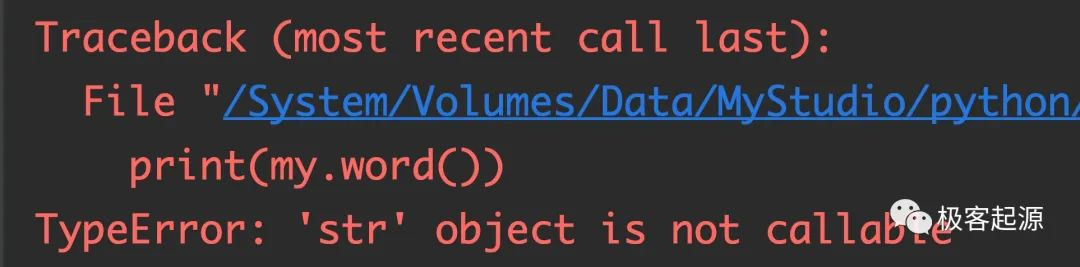## 2. property装饰器的原理``````print(property)
``````

``````<class 'property'>
``````

``````property(word)
``````

``````class MyClass:
def __init__(self, word):
self._word = word
@property
def word(self):
return self._word
# 输出被修饰的word方法的类型
print('word:', type(word))

def new_word(self):
return self._word
# 输出未被修饰的new_word方法的类型
print('new_word:', type(new_word))
new_word = property(new_word)
print(type(new_word))
my = MyClass("android")

print(my.word)
print(my.new_word)
``````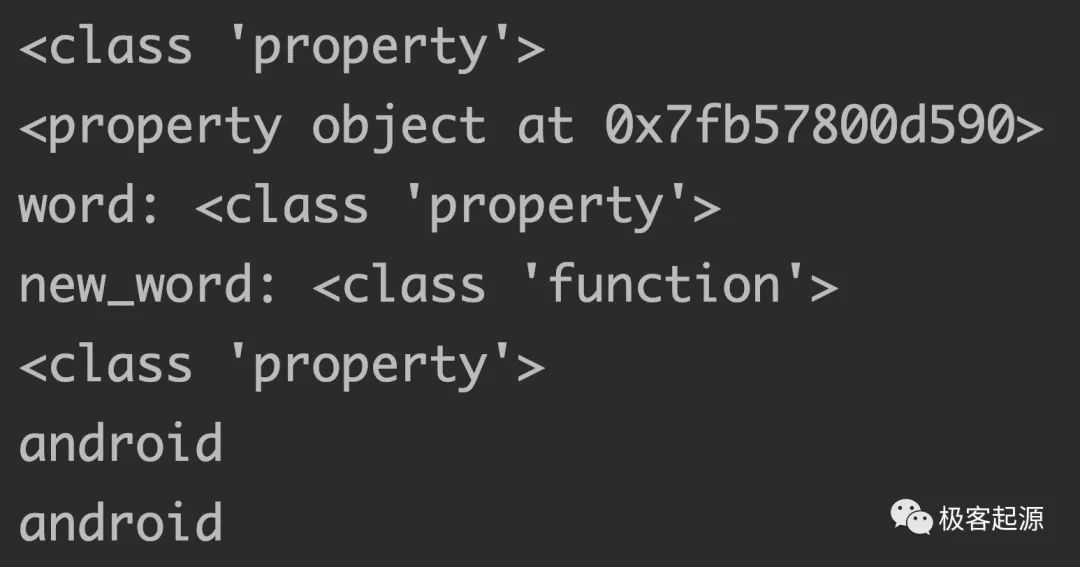``````@property
def new_word(self):
return self._word
``````

## 3. 让属性可写、可删除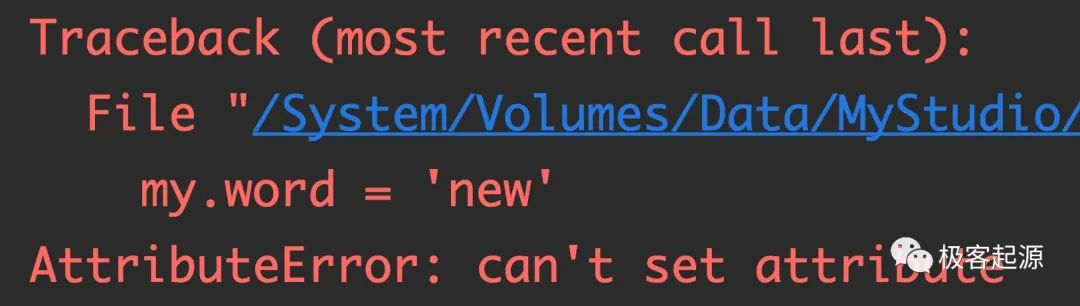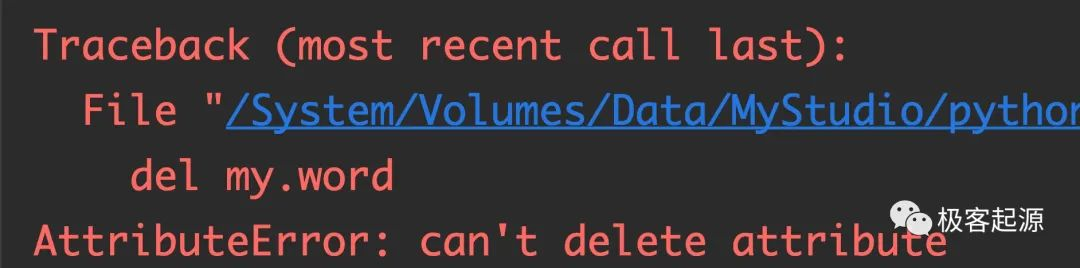``````class MyClass:
def __init__(self, word):
self._word = word
@property
def word(self):
return self._word
# 设置可写属性
@word.setter
def word(self, value):
self._word = value
# 设置可删除属性	，删除word属性时会调用该方法
@word.deleter
def word(self):
print('delete word')
self._word = ''
# 通过创建property实例的方式将new_word方法变成可读写和可删除的
def new_word(self):
return self._word
# 将new_word变成只读的属性，并且需要将property实例赋给一个新的变量，否则会被后面的new_word方法覆盖
new_word1 = property(new_word)
def new_word(self, value):
self._word = value
# 将new_word变成可写的属性
new_word1 = new_word1.setter(new_word)

def new_word(self):
print('delete new word')
# 将new_word变成可删除的属性
new_word = new_word1.deleter(new_word)

my = MyClass('hello')

print(my.word)
my.word = 'world'   #  def word(self, value):
print(my.word)
del my.word
print(my.word)

print('---------')
my = MyClass('ios')

print(my.new_word)
my.new_word = 'harmony'
print(my.new_word)
del my.new_word
print(my.new_word)
``````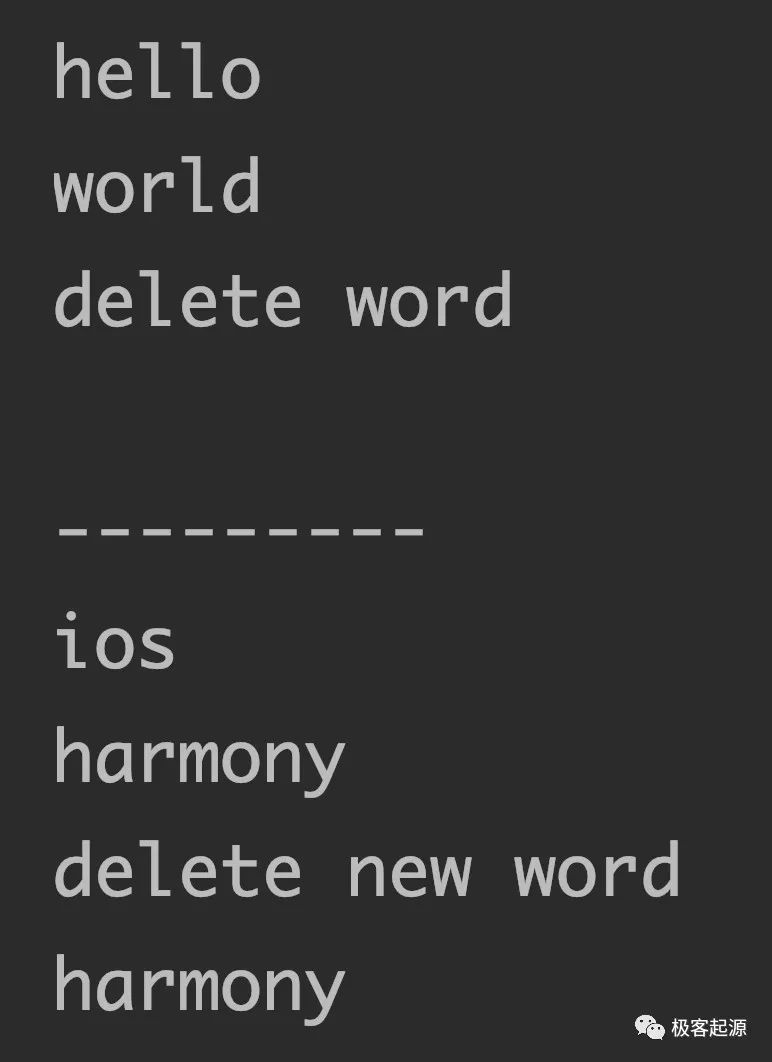## 4. 获得原始方法

(1)fget：获取被@property或@property.getter修饰的方法

(2)fset：获取被@property.setter修饰的方法

(3)fdel：获取被@property.deleter修饰的方法

``````class MyClass:
def __init__(self, word):
self._word = word
@property
def word(self):
return self._word
@word.setter
def word(self, value):
self._word = value
@word.deleter
def word(self):
print('delete word')
self._word = ''
fget_word = word.fget
fset_word = word.fset
fdel_word = word.fdel

my = MyClass('android')
print(my.fget_word())
my.fset_word('harmony')
print(my.fget_word())
print(my.fdel_word())
``````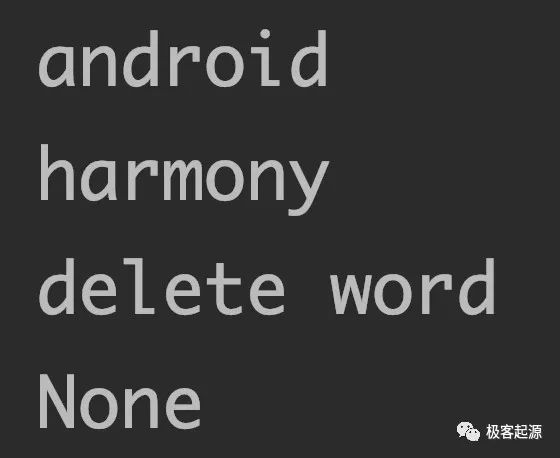Ω